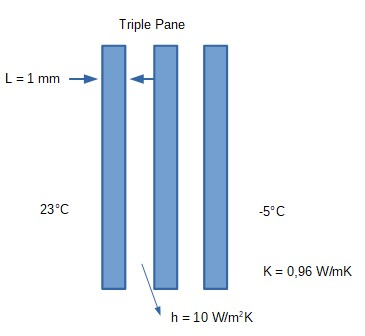# Consider the triple pane window shown, with three pieces of identical glass (Thickness of each...

## Question:

Consider the triple pane window shown, with three pieces of identical glass (Thickness of each glass = 1 mm and k = 0.96 W/mK) installed that seal the air in between. Heat transfer coefficient (h) in the sealed air is {eq}10 W/m^2K {/eq}. Determine the heat loss. N## Heat Transfer:

The heat transfer is the process in which the thermal energy gets transfer from a higher temperature to a lower temperature naturally and vice versa by doing some external work. The three modes of heat transfer are:

• Conduction
• Convection

Given Data

• Thickness of each glass is, {eq}t = 1\;{\rm{mm}} {/eq}.
• Thermal conductivity of glass is, {eq}k = 0.96\;{\rm{W/m}} \cdot {\rm{K}} {/eq}.
• Heat transfer coefficient is, {eq}h = 10\;{\rm{W/}}{{\rm{m}}^2} \cdot {\rm{K}} {/eq}.
• The temperature outside the window is, {eq}{T_1} = 23^\circ {\rm{C}} {/eq}.
• The temperature inside the window is, {eq}{T_2} = - 5^\circ {\rm{C}} {/eq}.

The total thickness due to three glasses is given as,

{eq}\begin{align*} {L_{total}} &= t + t + t\\ {L_{total}} &= 3\;{\rm{mm}} \end{align*} {/eq}

The heat transfer due to these glass plates is given as,

{eq}Q = \dfrac{{{T_1} - {T_2}}}{{\dfrac{1}{{{h_1}}} + \dfrac{1}{{{h_2}}} + \dfrac{{{L_{total}}}}{k}}} {/eq}

Substituting the values in above expression.

{eq}\begin{align*} Q &= \dfrac{{23 + 5}}{{\dfrac{1}{{10}} + \dfrac{1}{{10}} + \dfrac{{0.003}}{{0.96}}}}\\ Q &= 137.8\;{\rm{W/}}{{\rm{m}}^2} \end{align*} {/eq}

Thus, the heat loss is {eq}137.8\;{\rm{W/}}{{\rm{m}}^2} {/eq}.